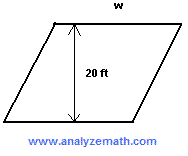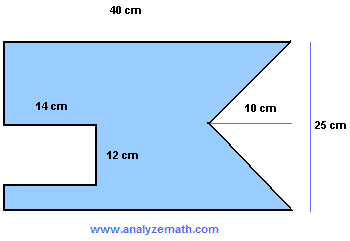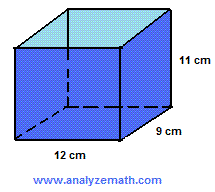# Grade 8 Geometry Problems and Questions with with Solutions and Explanations

Detailed solutions and full explanations to grade 8 math Grade 8 Geometry Problems and Questions are presented.

 Find the total surface area and the volume of a closed cylindrical container with radius 5 cm and a height of 34 cm. Solution The total surface area A of a cylinder is given by the sum of the area of the lateral surface plus the areas of the base and bottom of the container. A = 2 � radius � π � height + π � radius2 + π � radius2 = 2 � 5 � π � 34 + π � 52 + π � 52 = 340 π + 25 π + 25 π = 390 π square centimeters = 1224.6 square centimeters (using π = 3.14) The volume V of the given cylinder is equal to V = π � radius2 � height = π � 25 � 34 = 850 π cubic centimeters = 2669 cubic centimeters (using π = 3.14) Find the total surface area and the volume of a closed conical container with radius 5 cm and a height of 15 cm.(round your answer to the nearest unit.) Solution The total surface area A of a cone is given by the sum of the area of the lateral surface plus the area of the base. A = π � radius � slant height + π � radius2 The slant height S, the height of the cone (h = 15 cm) and the radius (r = 5 cm) of its base form a right triangle where S may be found using Pythagor's theorem as follows. S2 = 152 + 52 = 250 S = 15.8 cm A = π � 5 � 15.8 + π � 25 = 104 π square centimeters = 327 square centimeters (using π = 3.14) The volume V of the cone is given by. V = (1/3) � π � r2 � h = (1/3) � π � 52 � 15 = 125 π cubic centimeters = 393 cubic centimeters A cube has a total surface area of the six faces equal to 150 square feet. What is the volume of the cube? Solution The surface area of one square face is equal to 150 / 6 = 25 square feet Since the face of a cube is square, if x is the length of an edge of the cube, then x2 = 25 square feet and x = 5 feet The volume V of the cube is equal to V = 5 � 5 � 5 = 125 cubic feet Which two angles are complementary? 21 degrees and 78 degrees 58 degrees and 22 degrees 67 degrees and 23 degrees 140 degrees and 40 degrees Solution Two angles are complementary if the sum of their measures is equal to 90� 21 � + 78 � = 99 � 58 � + 22 � = 80 � 67 � + 23 � = 90 � 140 � + 40 � = 180 � Angles 67 � and 23 � are complementary Which two angles are not supplementary? 30 degrees and 150 degrees 5 degrees and 175 degrees 89 degrees and 91 degrees 23 degrees and 177 degrees Solution Two angles are supplementary if the sum of their measures is equal to 180� 30 � + 150 � = 180 � 5 � + 175 � = 180 � 89 � + 91 � = 180 � 23 � + 177 � = 200 � Angles 23 � and 177 � are not supplementary. Find the height h of the trapezoid so that its area is equal to 400 square cm.. Solution The area A of a trapezoid is given by the formula A = (1/2)(base1 + base2) � height = (1/2)(27 + 13) � h The area A of the trapezoid is equal to 400 square cm. Hence (1/2)(27 + 13) � h = 400 (1/2)(40) � h = 400 20 � h = 400 h = 20 cm Find the width w of the parallelogram so that its area is equal to 600 square feet.. Solution The area A of a parallelogram is equal to A = W � height = W � 20 = 600 W = 600 / 20 = 30 feet Find area of the shaded shape.. Solution The shaded (blue) shape is made up of a large rectangle of length 40 and width 25 from which a smaller rectangle (left) of width 14 and width 12 and a triangle (right) of base 25 and height 10 have been cut. Hence the area of this shape is equal to Area of large rectangle - area of smaller rectangle - area of triangle = 40 � 25 - 14 � 12 - (1/2) � 25 � 10 = 707 square cm Find the total surface area of the box open at the top.. Solution Area of the open box is equal to The areas of the front and back faces + the areas of the left and right faces + the area ot bottom = 2(12 � 11) + 2(9 � 11) + 9 � 12 = 570 square cm If the quadrilateral ABCD is a parallelogram, what are the coordinates of point D?. Solution Since ABCD is a parallelogram sides AB and DC are parallel. Because points A and B have the same y coordinate, AB is parallel to the x axis. Hence DC is also parallel to the x axis and therefore the y coordinate of point D is the same as the y coordinate of C and is equal to 2. Also distance AB must be equal to distance CD. Hence if a is the x coordinate of C, then 7 - a = 8 - 2 x = 1 Point C has coordinates (1 , 2) Which of these are right triangles?. Solution The unknown angle in triangle A) is equal to: 180 - (62 +28) = 90� Triangle A) is a right triangle. To be a right triangle, the sides of triangle B) must satisfy Pythagora's theorem. 32 + 3.92 ? = 52 left side: 32 + 3.92 = 24.21 right side: 52 = 25 Triangle B) is not a right triangle. To be a right triangle, the sides of triangle C) must satisfy Pythagora's theorem. 92 + 122 ? = 152 left side: 92 + 122 = 225 right side: 152 = 225 Triangle C) is a right triangle. Find x if triangle ABC is a right triangle with angle B = 90�.. Solution Angle BAC is equal to 180 - (47 + 90) = 43� ∠ABC + x + 35 = 180 x = 180 - (43 + 35) = 102� Find all the unknown sides x, y, z and w if all three triangles are similar.. Solution When two triangles are similar, their corresponding angles are equal and their corresponding sides are proportional. The corresponding sides of the triangle on the left and the one in the middle gives: 12 / 17 = 36 / y and 12 / 3 = 36 / x Solve the above equations to find y = 51 and x = 9 The corresponding sides of the triangle on the right and the one in the middle gives: 12 / 17 = z / 204 and 17 / 3 = 204 / w Solve the above equations to find z = 144 and w = 36 A quadrilateral with vertices (-2,6) , (6,8) , (9,2) and (4,-1) is reflected on the x axis. What are the coordinates of the vertices of the quadrilateral after reflection? Solution A reflection on the x axis of a point with coordinates (a , b) on the x axis gives the point (a , -b). Hence the 4 points are reflected into the points: (-2,-(6)) , (6,-(8)) , (9,-(2)) and (4,-(-1)) simplify to (-2,-6) , (6,-8) , (9,-2) and (4,1) The side of cube A is 3 times the side of cube B. The volume of cube A is 3,375 cubic feet. Find the volume of cube B. Solution Let x be the side of cube B. Hence the side of cube A is 3 x. The volume of cube A is given by Va = (3 x) � (3 x) � (3 x) Simplify the right side to obtain Va = 27 x 3 The volume of cube B is given by Vb = x � x � x = x 3 The ratio of Vb and Va is given by Vb / Va = x 3 / 27 x 3 Simplify to obtain Vb / Va = 1 / 27 Va is given, hence Vb / 3375 = 1 / 27 Multiply both sides by 3375 3375 � Vb / 3375 = 3375 � 1 / 27 Simplify to obtain Vb = 3375 / 27 = 125 cubic feet The length of rectangle A is 24 cm and the length of rectangle B is 96 cm. The two rectangles are similar. Find the ratio of the area of A to the area of B. Solution Let Wa and Wb be the width of rctangles A and B respectively. Since the two rectangles are similar, we have the following proportionality Wa / 24 = Wb / 96 The areas Aa and Ab of rectangles A and B respectively are given by Aa = 24 Wa and Wb = 96 Wb The ratio Aa / Ab is given by Aa / Ab = 24 Wa / 96 Wb = (24 / 96)� (Wa / Wb) The equation Wa / 24 = Wb / 96 obtained above may be written as Wa / Wb = 24 / 96 We now substitute to obtain Aa / Ab = (24 / 96) � (24 / 96) = 1 / 16 Answers to the Above Questions area = 390 π square cm , volume = 850 π cubic cm area = 327 square cm , volume = 393 cubic cm volume = 125 cubic feet C) 67 degrees and 23 degrees are complementary D) 23 degrees and 177 degrees are not supplementary h = 20 cm w = 30 feet area = 707 square cm surface area = 570 square cm (1 , 2) triangles A) and C) are right triangles x = 102 o x = 9 , y = 51 , z = 144 , w = 36 vertices after reflection (-2,-6) , (6,-8) , (9,-2) and (4,1) volume of cube B = 125 cubic feet ratio of area of A to area of B = 1:16